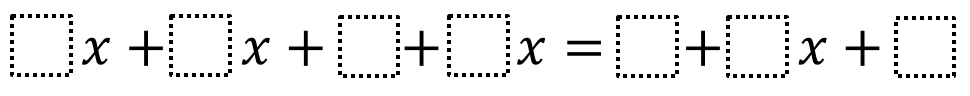# Equivalent Expressions 1

Directions: Using the digits 1 to 9 at most one time each, fill in the boxes to create two expressions that are equivalent to one another.### Hint

What values will make the constants equivalent? What values will make the variable terms equivalent?

Here are some possibilities:
1x + 2x + 9 + 3x = 4 + 6x + 5;
1x + 2x + 8 + 4x = 3 + 7x + 5

Source: Will Case

## Greatest Common Factor

Directions: Using the digits 0 to 9 at most one time each, place a digit …

1.2.8x+5x+2+4x=4+5x+9

3.4.5.5x + 3x + 4 + 1x = 3 + 9x + 1

•I changed my answer. 4x + 2x + 8 + 3x = 7 + 9x + 1

6.7.8.